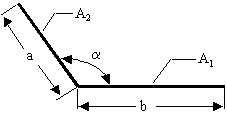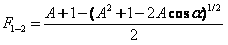Cody

# Problem 2807. Radiation Heat Transfer — View Factors (3)

Created by goc3 in Community

View factors (aka configuration factors) are utilized in some radiation heat transfer models to estimate heat transfer rates between surfaces. In particular, the thermal energy leaving a given surface is applied to other surfaces, as appropriate, based on how much the hot surface "sees" the other surfaces. As such, view factors are purely geometrical in nature. A range of view factor formulae are available here.

For this problem, calculate the view factor from surface 1 to surface 2 (F_1-2) for two infinitely long plates of different finite widths and having a common edge given the angle between the plates:-Any of the variables can be a vector. Also, note that A = a/b, and is different than A_1 or A_2. Finally, the angle is provided in degrees.

### Solution Stats

39.13% Correct | 60.87% Incorrect
Last solution submitted on May 15, 2019